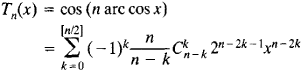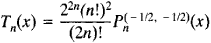# Chebyshev Polynomials

(redirected from Chebyshev form)

## Chebyshev polynomials

[′cheb·ə·shəf ‚päl·i′nō·mē·əlz]
(mathematics)
A family of orthogonal polynomials which solve Chebyshev's differential equation.
McGraw-Hill Dictionary of Scientific & Technical Terms, 6E, Copyright © 2003 by The McGraw-Hill Companies, Inc.
The following article is from The Great Soviet Encyclopedia (1979). It might be outdated or ideologically biased.

## Chebyshev Polynomials

Chebyshev polynomials of the first kind are a special system of polynomials of successively increasing degree. For n = 0, 1, 2, . . . they are defined by the formulaIn particular, T0 = 1, T1 = x, T2 = 2x2 – 1, T3 = 4x3 – 3x and T4 = 8x4 – 8x2 + 1.

The polynomials Tn (x) are orthogonal with respect to the weight function (1 – x2)–½ on the interval [–1, +1] (seeORTHOGONAL POLYNOMIALS). They satisfy the differential equation

(1 – x2)yxy + n2y = 0

and the recursion formula

Tn+1 (x) = 2xTn(x) – Tn – 1(x)

Chebyshev polynomials of the first kind are a special case of the Jacobi polynomials Pn(α,β)(x):Chebyshev polynomials of the second kind Un (x) are a system of polynomials that are orthogonal with respect to the weight function (1 – x2)½ on the interval [–1, +1]. The relation between Chebyshev polynomials of the second kind and Chebyshev polynomials of the first kind is given by, for example, the recursion formula

(1 – x2)Un – 1 (x) = xTn (x) – Tn+l(x)

### REFERENCES

Chebyshev, P. L. Poln. sobr. soch., vols. 2–3. Moscow-Leningrad, 1947–48.
Szegö, G. Ortogonal’nye mnogochleny. Moscow, 1962. (Translated from English.)
The Great Soviet Encyclopedia, 3rd Edition (1970-1979). © 2010 The Gale Group, Inc. All rights reserved.
Site: Follow: Share:
Open / Close